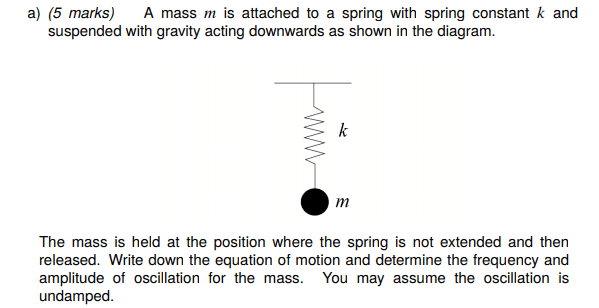# Equation of motion and normal modes of a coupled oscillator

Homework Statement:
Find the equation of motion of a mass on a spring
Relevant Equations:
x(t) = Acos(ωt + φ)
This is a question from an exercise I don't have the answers to.

I have been trying to figure this out for a long time and don't know what to do after writing

mx''¨(t)=−kx(t)+mg
I figure that the frequency ω=√(k/m) since the mg term is constant and the kx term is the only term that changes.

I have the equation of motion written as x(t) = Acos(ωt + φ) but do not know how to go about finding the amplitude either.

Any help would be great thanks!